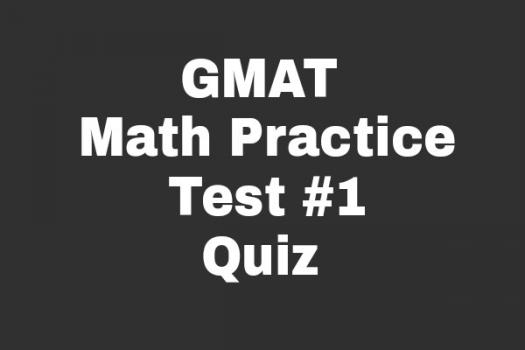# GMAT Math Practice Test

10 Questions | Total Attempts: 123SettingsGet drilled on Pythagoras's law, probability laws and simple arithmetic laws in this quiz. These are essential in solving real life problems available in mathematically oriented disciplines. By this standard, hone your speed and accuracy skill as you prepare for GMAT Exam. Would you like to give it a shot?

Related Topics
• 1.
What is the 75% of 200?
• A.

170

• B.

150

• C.

190

• D.

120

• 2.
Which of these is a perfect square?
• A.

400

• B.

200

• C.

100

• D.

300

• 3.
How many days are in an ideal year?
• A.

230 days

• B.

365 days

• C.

287 days

• D.

375 days

• 4.
How many time can 12 be gotten in 60?
• A.

4 times

• B.

9 times

• C.

7 times

• D.

5 times

• 5.
What is x in this equation; x + 9 = 15
• A.

12

• B.

6

• C.

10

• D.

8

• 6.
What is result of this expression; 1/2 of 20 ?
• A.

19.5

• B.

19

• C.

10

• D.

9

• 7.
What comes first in the order of a mathematical operation?
• A.

Of

• B.

Multiplication

• C.

Division

• D.

Bracket

• 8.
What is the result of deducting 12 from 60?
• A.

48

• B.

23

• C.

18

• D.

42

• 9.
What is the total of these numbers; 2, 5 , 7, 9, 3?
• A.

30

• B.

33

• C.

39

• D.

26

• 10.
How many times can 2 be obtained in 200?
• A.

12 times

• B.

29 times

• C.

23 times

• D.

100 times